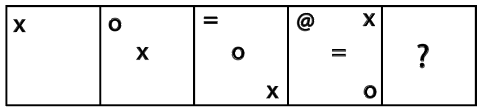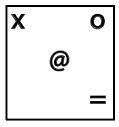Question 4

# Select the figure that can replace the question mark (?) in the following series.Solution

Given figurethe above image follows the same pattern is given belowtherefore? = (B) Ans

• Free SSC Study Material - 18000 Questions
• 230+ SSC previous papers with solutions PDF